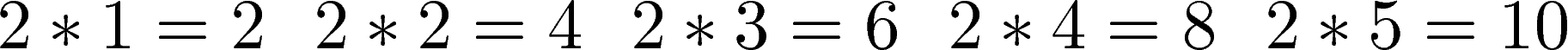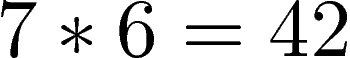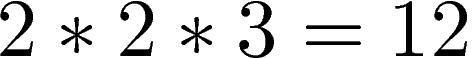# Basic Algebraic Concepts

This post will provide insights into some basic algebraic concepts. Such information is actually useful for people who are doing research but may not have the foundational mathematical experience.

MultipleA multiple is a product of  and a counting number of n. In the preceding sentence, we actually have two unknown values which are.

• n
• Counting number

The can be any value, while the counting number usually starts at 1 and continues by increasing by 1 each time until you want it to stop. This is how this would look if we used the term n,  counting number, and multiple of n.

n * counting number = multiple of n

For example, if we say that = 2 and the counting numbers are 1,2,3,4,5. We get the following multiples of 2.You can see that the never changes and remains constant as the value 2. The counting number starts at 1 and increases each time. Lastly, the multiple is the product of n and the counting number.

Let’s take one example from above

2 * 3 = 6

Here are some conclusions we can make from this simple equation

• 6 is a multiple of 2. In other words, if I multiply 2 by a certain counting number I can get the whole number of 6.
• 6 is divisible by 2. This means that if I divide 2 into six I will get a whole number counting number which in this case is 3.

Divisibility Rules

There are also several divisibility rules in math. They can be used as shortcuts to determine if a number is divisible by another without having to do any calculation.

A number is divisible by

• 2 when the last digit of the number 0, 2, 4, 6, 8
• Example 14, 20, 26,
• 3 when the sum of the digits is divisible by 3
• Example 27 is divisible by 3 because 2 + 7 = 9 and 9 is divisible by 3
• 5 when the number’s last digit is 0 or 5
• Example 10, 20, 25
• 6 when the number is divisible by 2 and 3
• Example 24 is divisible by 6 because it is divisible by 2 because the last digit is for and it is divisible by 3 because 2 + 4 = 6 and six is divisible by 3
• 10 when the number ends with 0
• Example 20, 30 , 40, 100

Factors

Factors are two or more numbers that when multiplied produce a number. For exampleThe numbers 7 and 6 are factors of 42. In other words, 7 and 6 are divisible by 42. A number that has only itself and one as factors is known as a prime number. Examples include 2, 3, 5, 7, 11, 13. A number that has many factors is called a composite number and includes such examples as 4, 8, 10, 12, 14.

An important concept in basic algebra is understanding how to find the prime numbers of a composite number. This is known as prime factorization and is done through the development of a factor tree. A factor tree breaks down a composite number into the various factors of it. These factors are further broken down into their factors until you reach the bottom of a tree that only contains prime numbers. Below is an exampleYou can see in the tree above that the prime factors of 12 are 2 and 3. If we take all of the prime factors and multiply them together we will get the answer 12.Conclusion

Understanding these basic terms can only help someone who maybe jumped straight into statistics in grad school without have the prior thorough experience in basic algebra.